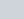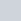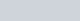are zeroes of the polynomial x2 − 2x − 15,
Question:

If $\alpha, \beta$ are zeroes of the polynomial $x^{2}-2 x-15$, then form a quadratic polynomial whose zeroes are $(2 \alpha)$ and $(2 \beta)$.

Solution:

The given polynomial is $P(x)=x^{2}-2 x-15$ and $\alpha$ and $\beta$ are the zeroes of the polynomial $P(x)$, then we have to find another polynomial whose zeroes are $2 \alpha, 2 \beta$

Now we comparing the given polynomial $P(x)$ with $a x^{2}+b x+c$, we get

$a=1, b=-2$, and $c=-15$

We know that

$\alpha+\beta=-\frac{b}{a}$

$=-\frac{-2}{1}=2$

$\alpha \beta=\frac{c}{a}$

$=\frac{-15}{1}=-15$

The polynomial whose zeroes are,is

$P(x)=x^{2}-(2 \alpha+2 \beta) x+2 \alpha .2 \beta$

$=x^{2}-2(\alpha+\beta) x+4 \alpha \beta$

$=x^{2}-2 \times 2 x+4 \times(-15)$

$=x^{2}-4 x-60$

Hence the polynomial is